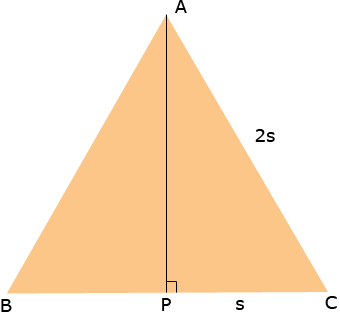Subject: height of a triangle as a function Name: Ryan Who are you: Student Hi, this is the question I have: Let 2s denote the length of the side of an equilateral triangle. Express the height of the triangle as a function of s Hi Ryan, Draw a diagramThe length of AP is the height of the triangle and by the symmetry the lengths of BP and PC are equal. Thus the length of PC is s. APC is a right triangle. What does Pythagoras Theorem tell you about the length of AP? Penny